# Bessel Functions

One of the varieties of special functions which are encountered in the solution of physical problems is the class of functions called Bessel functions. They are solutions to a very important differential equation, the Bessel equation: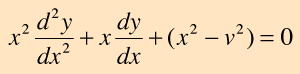The solutions to this equation are in the form of infinite series which are called Bessel funtions of the first kind. The expression for the sum is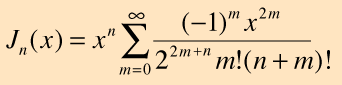Values for the Bessel functions can be found in most collections of mathematical tables. Bessel functions are encountered in physical situations where there is cylindrical symmetry. This occurs in problems involving electric fields, vibrations, heat conduction, optical diffraction and others.

For the specific application to a spherical potential well in quantum mechanics, another form called spherical bessel functions appears.

Index

Reference
Kreyszig
Sec 4.5

 HyperPhysics*****HyperMath*****Calculus R Nave
Go Back

# Spherical Bessel Functions

A specific class of special functions called spherical Bessel functions arises in problems of spherical symmetry like the spherical potential well in quantum mechanics. The first three forms are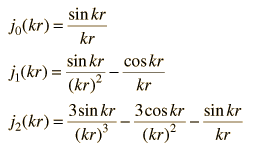From the first form, the higher order forms can be generated from the relationship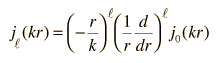It is sometimes useful to have the limiting cases for these functions for very large or very small distances: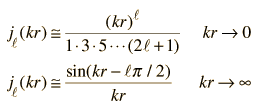Index

Reference
Krane
Ch 2

 HyperPhysics*****HyperMath*****Calculus R Nave
Go Back